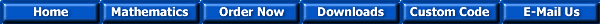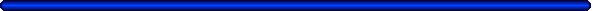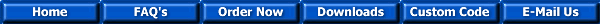The SuperTable® Add-in for Microsoft Excel XP and Excel 2003The Mathematics Behind the AACode SuperTable® Add-in

The AACode® SuperTable® Add-in gives you incredible number crunching power!

You don't need to understand how AACode SuperTable® functions and macros work to use them.  You are almost always furnished with only a few dots on a graph, several discrete values in a table, or a limited amount of measured information. The question is, how can you predict mathematical values at points other that those you already know?

AACode SuperTable® functions and macros provide powerful, convenient, and easy to use solutions inside your Microsoft Excel spreadsheets!

The AACode SuperTable® functions; VInterp, HInterp, VSpline, HSpline, VPolyfit and HPolyfit all work the same way as the as the standard Excel functions Vlookup and Hlookup.  All you need to specify is the data value to lookup, the range the data can be found in, and the number of offset rows or columns from the first line of the table, to the row or column where the desired data values are.  The first row or column of data has to be in ascending order and the value to be looked up has to be in the range of your data. Within these limits, AACode SuperTable® functions instantly return the interpolated answer you are looking for.  They can be nested with other Excel functions, inside formulae, and copied at will.

Once you realize just how useful, easy to use, and powerful AACode SuperTable® functions are; you won't be able to write an Excel Spreadsheet without them!

With the macros VSplineCoef, HSplineCoef, VPolyfitCoef, and HPolyfitCoef you can generate an equation that you can nest in your Excel Spreadsheets and completely eliminate the need for the original lookup table!  With the macro VRegressCoef and HRegressCoef you can test your data to determine the most accurate AACode SuperTable® function or macro to use.  If you work with electrical circuits, finite element analysis and similar matrix based mathematical problems you can use the AACode SuperTable® macro SimLinEqu to automatically calculate the solution for up to fifty simultaneous linear equations with fifty unknowns using Matrix Mathematics.

The AACode SuperTable® Add-in functions VInterp, HInterp, VSpline, HSpline, VPolyfit and HPolyfit correct a fundamental problem with the standard Excel functions Vlookup and Hlookup.  Vlookup and Hlookup do not correctly lookup intermediate values in a data set.  Instead, they use the nearest value entered in the data table as the lookup value.  Using Vlookup or Hlookup you might find unacceptable errors in your calculations, especially if the data you have is widely spaced or non-linear.  AACode SuperTable® corrects this problem with accurate and automatic mathematical interpolation.  With the AACode SuperTable® Add-in functions VInterp, HInterp, VSpline, HSpline, VPolyfit and HPolyfit you instantly receive the intermediate value answers you want.

AACode SuperTable® Functions Include:

VInterp and HInterp automatically perform linear interpolation on your data.  They are ideal for use with closely spaced or basically linear data sets.

Examples:  Generation of intermediate data to fill in cells in lookup tables or stepwise computations, interpolation of material properties tables, distribution of costs to multiple accounts, and interpolation of tax or labor rate tables.

VSpline and HSpline automatically perform spline interpolation using adjacent cubic polynomials to smoothly connect one point to the next, giving better interpolations for curved functions.  Because of the number of calculations involved, they are relatively slow for large data sets but are great when linear interpolation is not accurate enough.

Examples:  Interpolation of position data of a body subject to random forces (a flight model) or material properties that vary non-linearly and filling in gaps in sampled process or sensor data.

The Macros VSplineCoef and HSplineCoef and their corresponding functions VLSpline and HLSpline perform the same calculation that VSpline and HSpline do, they just work faster.  The computationally intensive process of generating the spline polynomial coefficients is done only once by running the Macros VSplineCoef or HSplineCoef on your data set.  Because the functions VLSpline and HLSpline work faster, they are better suited to applications where calculation time is limited.

Examples:  Rapid interpolation of large data tables, on line process control, interpretation of data acquisition information, and sensor output linearization.

VPolyfit and HPolyfit automatically calculate the best fit polynomial of the order you specify for the data set you select.  The result is a an algorithm that represents the functional relationship of the data, but does not necessarily pass through each point.

Examples:  Analysis of measured process values, prediction of physical values from measured table information, computational geometry, and precision fitting of experimental data.

The Macros VPolyfitCoef and HPolyfitCoef perform the same calculation that VPolyfit and HPolyfit do except they return a formula that can be pasted on any Excel spreadsheet.  You can condense a huge lookup table into a single algorithm!

Examples:  Reduction of physical property tables for use in process control correction, Inbetween animation frames, key frame animation, on-line process measurement, display of process values, geometric transformation, formula derivation from raw data, process control, sensor characterization.

The Macros VRegressCoef and HRegressCoef automatically calculate the Linear, Exponential, Power, and Logarithmic regressions and their respective Coefficients of Determination.  Each regression can be run separately or all at once.  The equations generated can be cut and pasted into any Excel spreadsheet.  If the data follows one of the standard regressions, the macro returns the simplest and fastest running algorithm.

Examples:  Optimum fitting of data sets and formula derivation from raw data.

The Macro SimLinEqu automatically calculates the solution for up to fifty simultaneous linear equations with fifty unknowns using Matrix Mathematics.  If the equations have no unique solution, the macro outputs "indeterminate" in place of the values of the unknowns.  The Macro SimLinEquCondNum is the same as SimLinEqu except it writes an additional table that can be used to determine how sensitive the results are to small changes in the coefficients.

Example:  Electronic circuit design, finite element analysis, heat transfer, structural calculations and algebraic mathematics.

The AACode SuperTable® Add-in will become a must-have addition to Microsoft Excel once you have a chance to use it on your tables and data.  It allows you to quickly analyze and interpret tables of data, find trends in accounting information, reduce scientific information to algorithms, project future sales, discover relationships in economic indexes, and lookup engineering properties automatically.  You will find yourself using formulas and making calculations you previously considered impractical.  AACode® functions work as easily as standard Excel functions like HLookup and VLookup.  On numerical data they are simply more powerful.  AACode SuperTable® MACROS are easy to use.  They are very similar to the Add-ins included by Microsoft as part of Excel.  We invite you to download a free copy of our AACode SuperTable® Add-in for evaluation.  You won't be disappointed.

Some useful references on standard functions in Excel are:www  http://www.aacode.com